# Future Value (FV)

Updated June 23, 2021

## What is Future Value (FV)?

Future value (FV) refers to a method of calculating how much the present value (PV) of an asset or cash will be worth at a specific time in the future.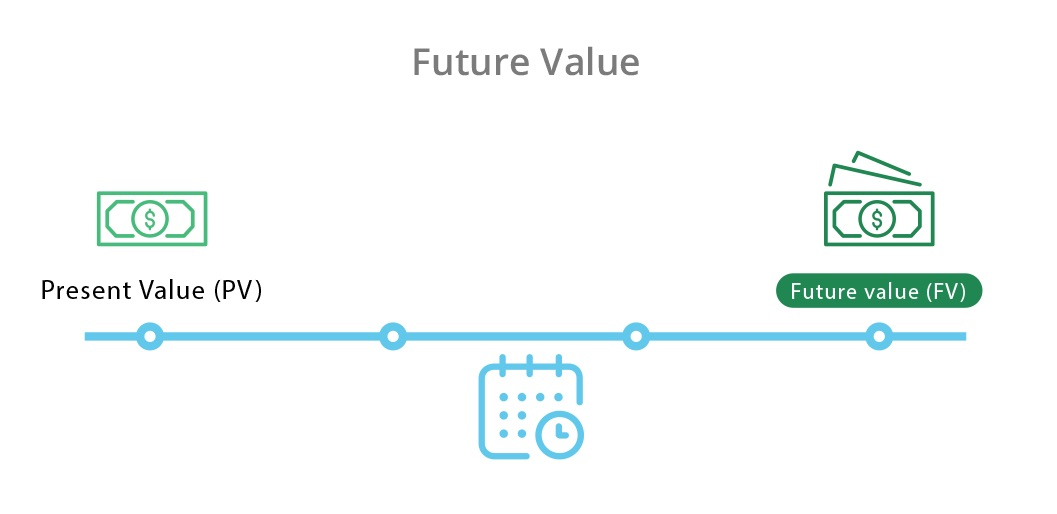## The Importance of Future Value

One dollar put into a savings account today might be worth more than one dollar a year from now. How does that work? The bank pays interest and your dollar earns money for that year. Thus, a dollar deposited today has a higher future value – the same is true for investments.

Future value is an important calculation because it tells investors and individuals how much an investment made today (present value) will be worth in the future.

### Limitations of Future Value

Although calculating future value has its benefits, future value does not include the following:

Each of these elements will affect the true value of money (or assets) in the future. It’s important to remember that future value is an estimation, not a guaranteed metric.

## The Future Value Formula

The future value formula requires three numbers:

• Present value, or how much the asset or cash is worth now (PV)

• What the annual interest rate is (r)

• Length of time/how many years the assets or cash will be left (n)

In a formula, it looks like this: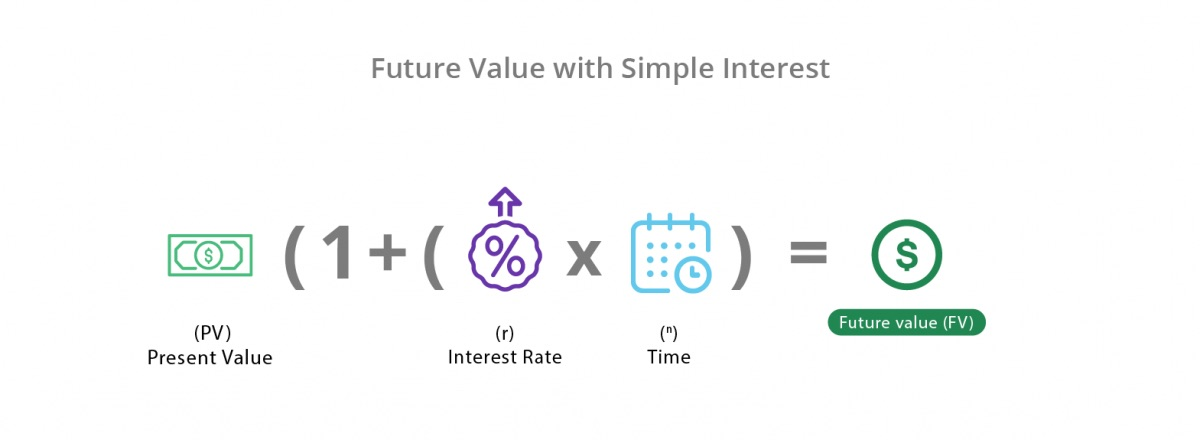However, if you’d like to calculate future value based on a monthly interest rate, R should be the monthly rate and N should be the number of months the value will be left to compound.

## Future Value Examples

There are two ways of calculating future value:

Simple interest is always based on the present value, whereas compounded interest means that the present value grows exponentially each year.

The future value formula changes slightly, depending on which calculation is carried out.

### Future Value with Simple Interest

Future value with simple interest uses the following formula:

Future Value = Present Value (1 + (Interest Rate x Number of Years))

Let’s say Bob invests \$1,000 for five years with an interest rate of 10%. The future value would be \$1,500.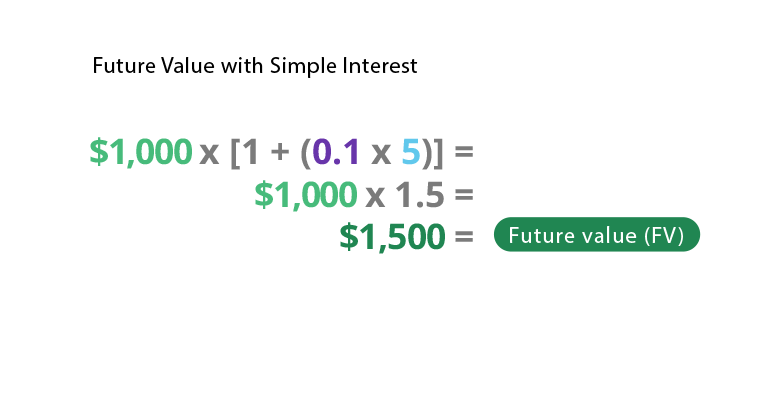### Future Value with Compound Interest

Future value with compounded interest needs to account for exponential growth (since interest collects on interest). The future value formula with compound interest looks like this:

Future Value = PV (1 + Annual Interest Rate) Number of Years

Let’s say Bob invests \$1,000 for five years with an interest rate of 10%. This time, it’s compounded annually. The future value of Bob’s investment would be \$1,610.51.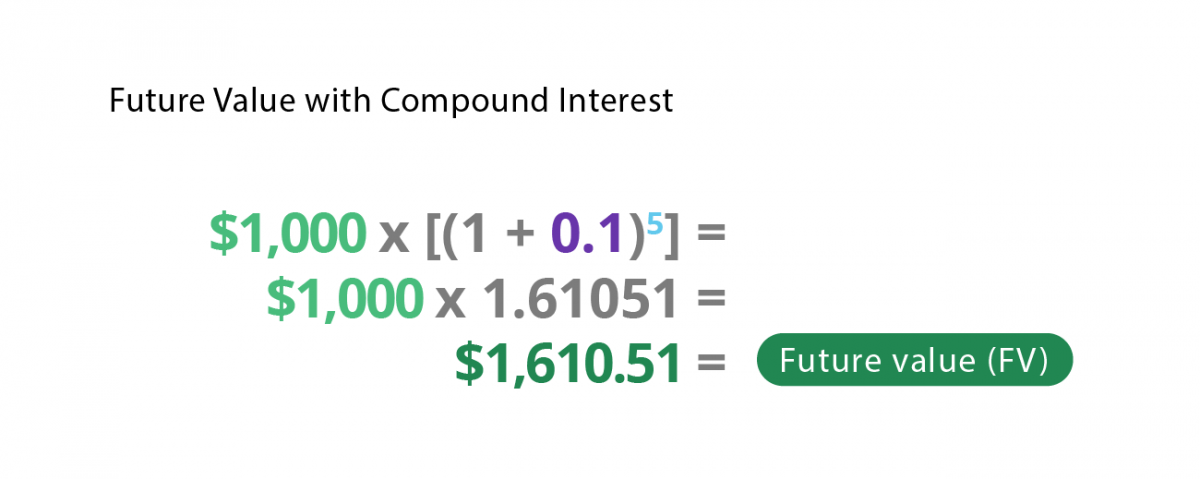When comparing the two future value calculations, consider the higher return on investment (ROI) seen with compound interest vs. simple interest.  To discover more on the power of compound interest, check out CDs and the Money Making Magic of Compound Interest.

### How to Calculate Future Value in Excel

If you are calculating compound interest, it can be much easier to find future value using an Excel spreadsheet (rather than running the calculation manually). To use an Excel spreadsheet, you can run the following formula:

=FV(rate,nper,pmt,pv,type)

Let’s use the annual compounding investment example above. It looks like this using the excel =FV function.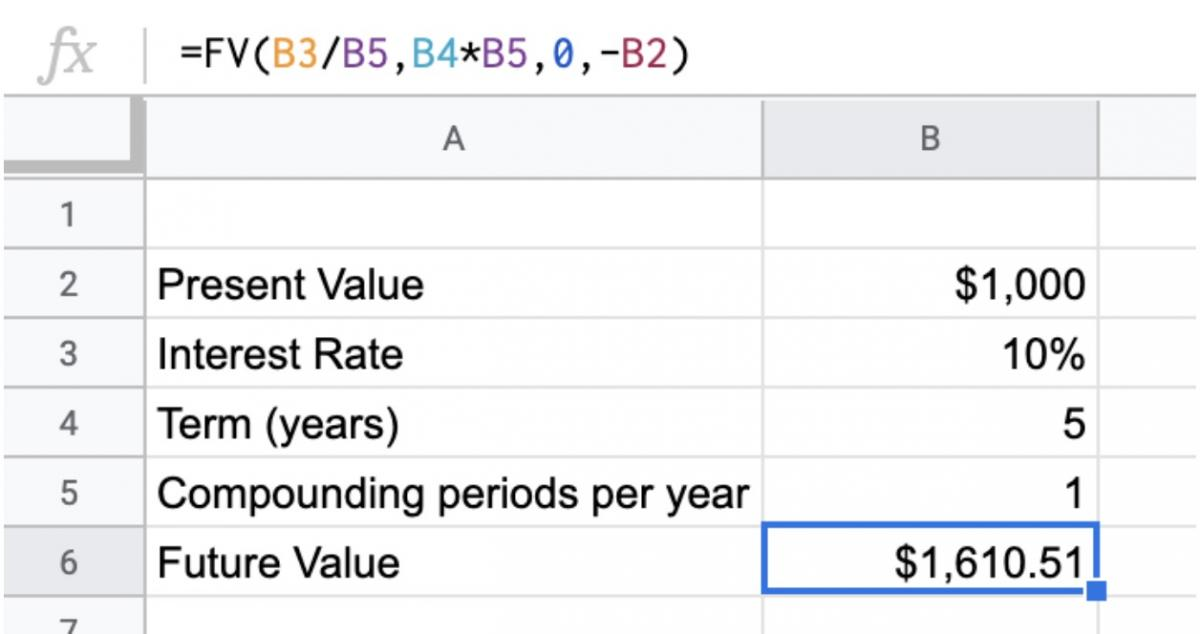## Future Value and Financial Planning

Future value allows you to analyze an investment’s possible return. You can also use future value to help build a savings plan for a specific amount in the future.

All of our content is verified for accuracy by Victoria Chan, CFA and our team of certified financial experts. We pride ourselves on quality, research, and transparency, and we value your feedback. Below you'll find answers to some of the most common reader questions about Future Value (FV).
##### Be the first to ask a question

Victoria Chan, CFA - 153Victoria Chan, CFACFA Charterholder

Chartered Financial Analyst

Vicky has over 10 years' experience in financial services, working in strategy and management consulting. She has also served as the deputy head of research for citibank's consumer bank in Australia, advising financial planners and clients on investment strategies and asset allocation.